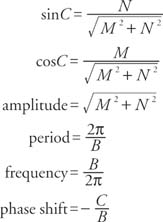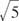## The Expression M sin Bt + N cos Bt

The equation y = M sin Bt + N cos Bt and the equation y = A sin (Bt + C) are equivalent where the relationships of A, B, C, M, and N are as follows. The proof is direct and follows from the sum identity for sine. The following is a summary of the properties of this relationship.

M sin Bt + N cos Bt =sin (Bt + C) given that Cis an angle with a point P(M, N) on its terminal side (see Figure 1 ).Figure 1
Reference graph for y = M sin Bt + N cos Bt.Example 1: Convert the equation y =sin 3 t + 2 cos 3 t to the form y = A sin (Bt + C). Find the period, frequency, amplitude, and phase shift (see Figure  2).Figure 2

Drawing for Example 1.Example 2: Convert the equation y = −sin π t + cos π t to the form y = A sin (Bt + C). Find the period, frequency, amplitude, and phase shift (see Figure 3 ).Figure 3
Drawing for Example 2.